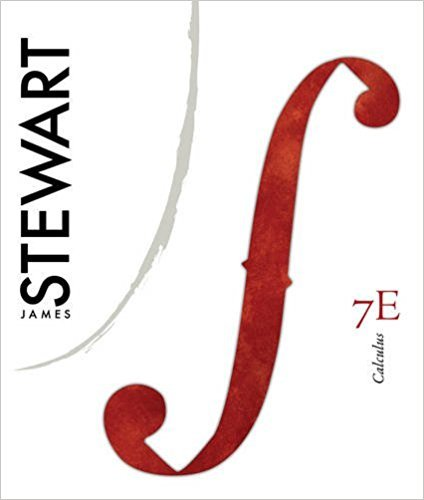×
Get Full Access to Calculus, - 7 Edition - Chapter 2 - Problem 61
Get Full Access to Calculus, - 7 Edition - Chapter 2 - Problem 61

×

# Find in terms of .fx x 2 tx fxISBN: 9780538497817 125

## Solution for problem 61 Chapter 2

Calculus, | 7th Edition

• Textbook Solutions
• 2901 Step-by-step solutions solved by professors and subject experts
• Get 24/7 help from StudySoup virtual teaching assistantsCalculus, | 7th Edition

4 5 1 400 Reviews
15
0
Problem 61

Find in terms of .fx x 2 tx fx

Step-by-Step Solution:
Step 1 of 3

Step 2 of 3

Step 3 of 3

##### ISBN: 9780538497817

This full solution covers the following key subjects: Find, terms. This expansive textbook survival guide covers 17 chapters, and 887 solutions. Since the solution to 61 from 2 chapter was answered, more than 287 students have viewed the full step-by-step answer. The full step-by-step solution to problem: 61 from chapter: 2 was answered by , our top Calculus solution expert on 11/10/17, 05:23PM. This textbook survival guide was created for the textbook: Calculus,, edition: 7. The answer to “Find in terms of .fx x 2 tx fx” is broken down into a number of easy to follow steps, and 9 words. Calculus, was written by and is associated to the ISBN: 9780538497817.

Unlock Textbook Solution